# RD Sharma Solutions For Class 12 Maths Exercise 1.2 Chapter 1 Relation

In this exercise, students will mainly get conceptual knowledge about equivalence relations. A relation is said to be an equivalence relation if it is reflexive, symmetric and transitive. Class 12 is a major turning point for the students to help them shape and achieve their future goals. The solutions for these problems are prepared in a comprehensive manner, which improves the problem-solving capacity of students. Exercise 1.2 of Chapter 1 Relations are available here in PDF format. To know more about these concepts, students can access RD Sharma Solutions for Class 12 Maths.

## Download the PDF of RD Sharma Solutions For Class 12 Chapter 1 – Relations Exercise 1.2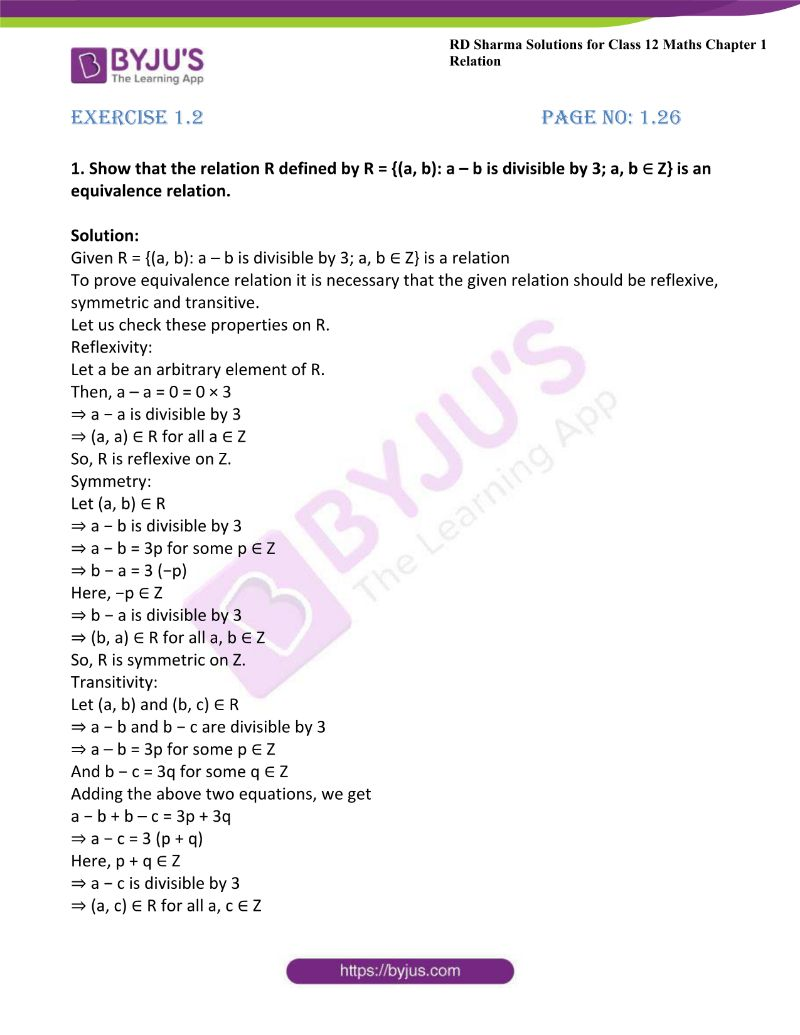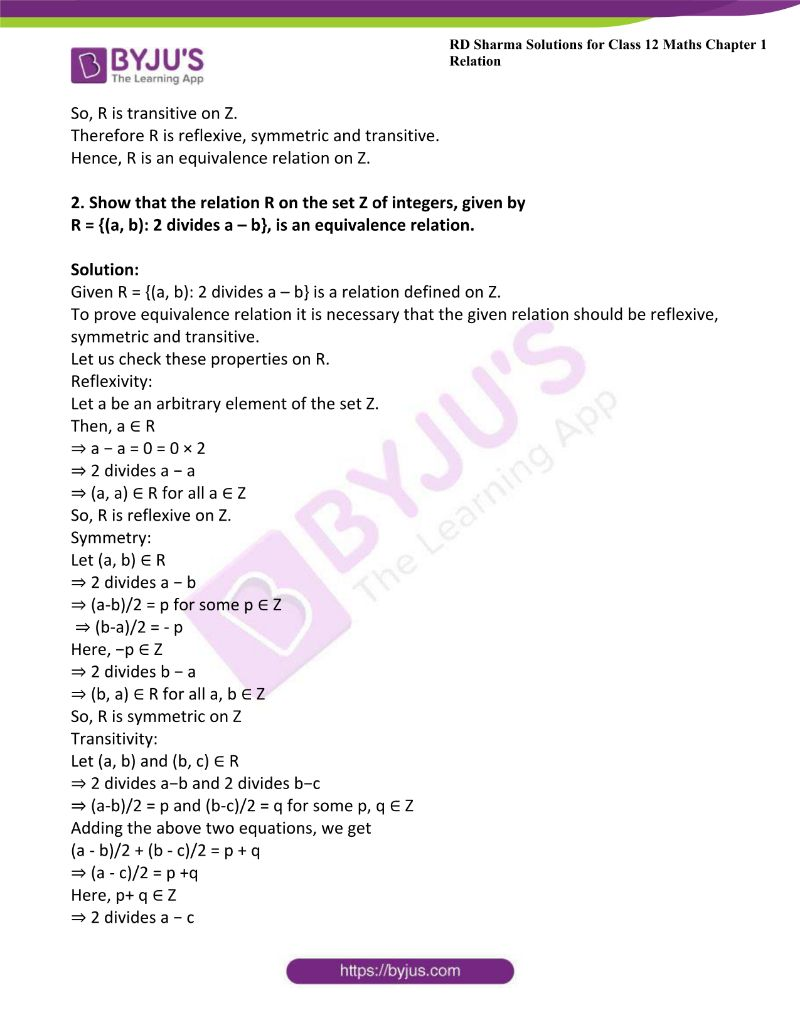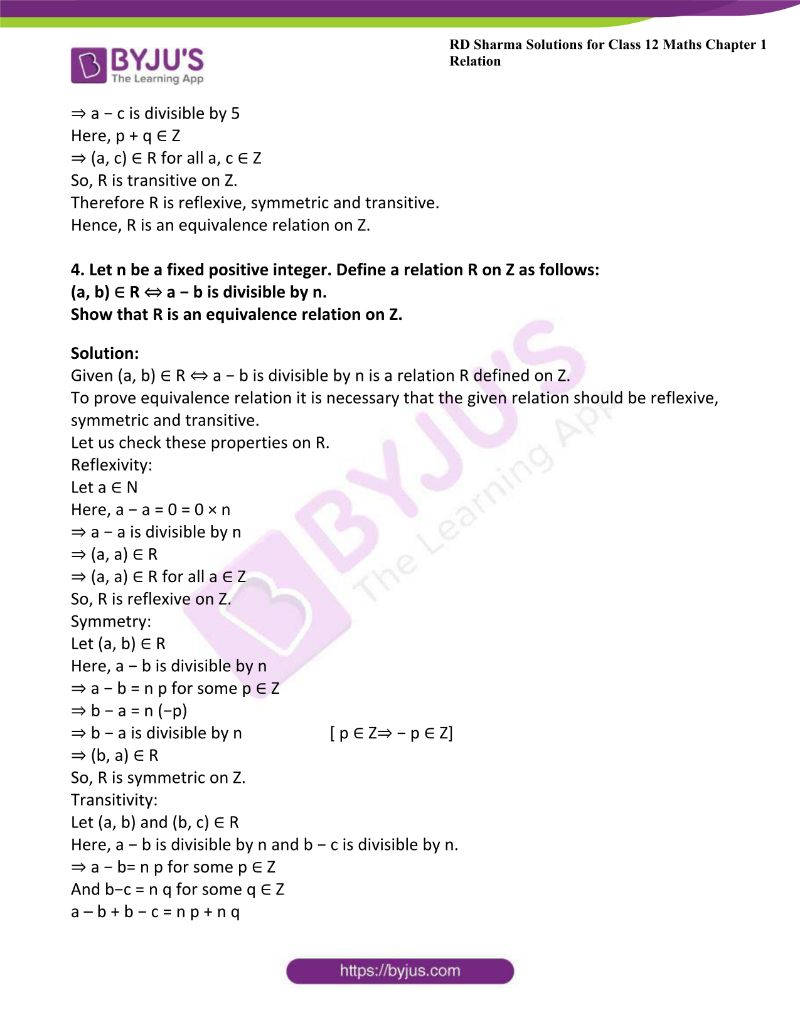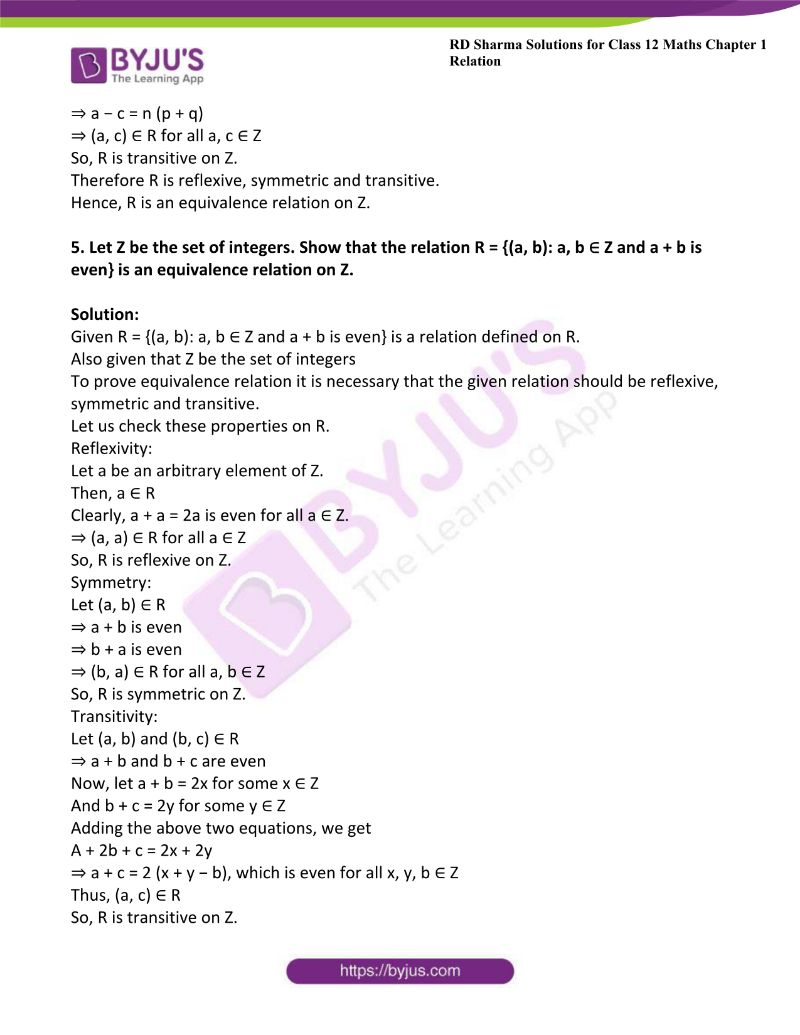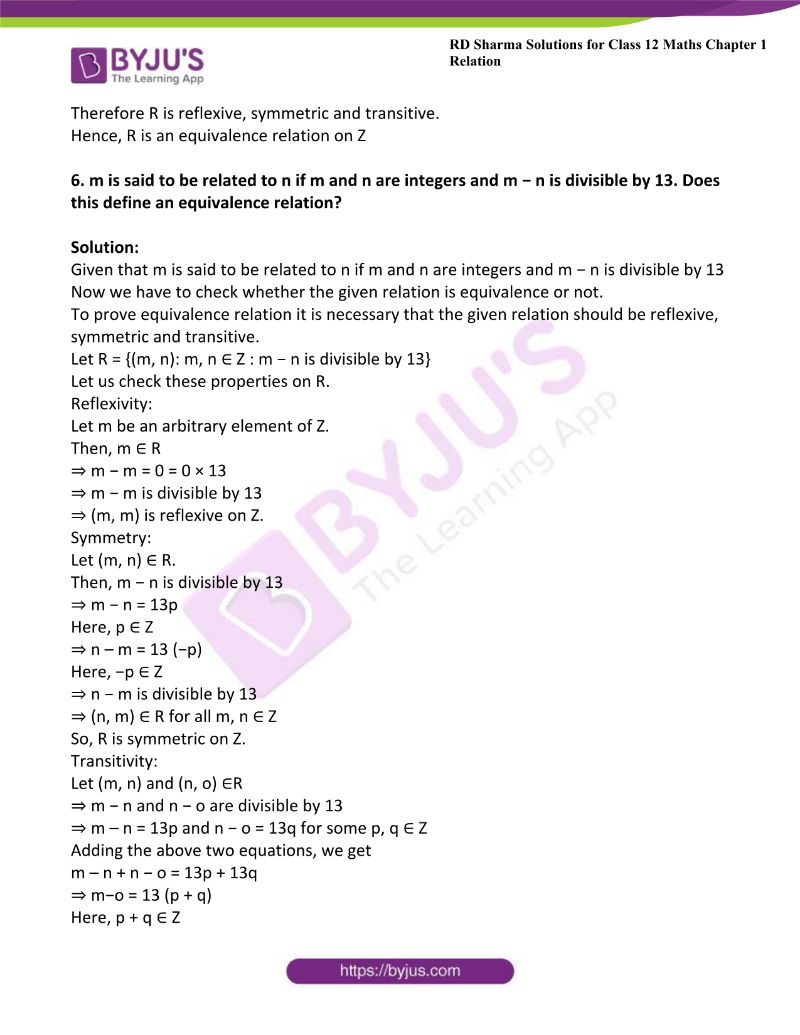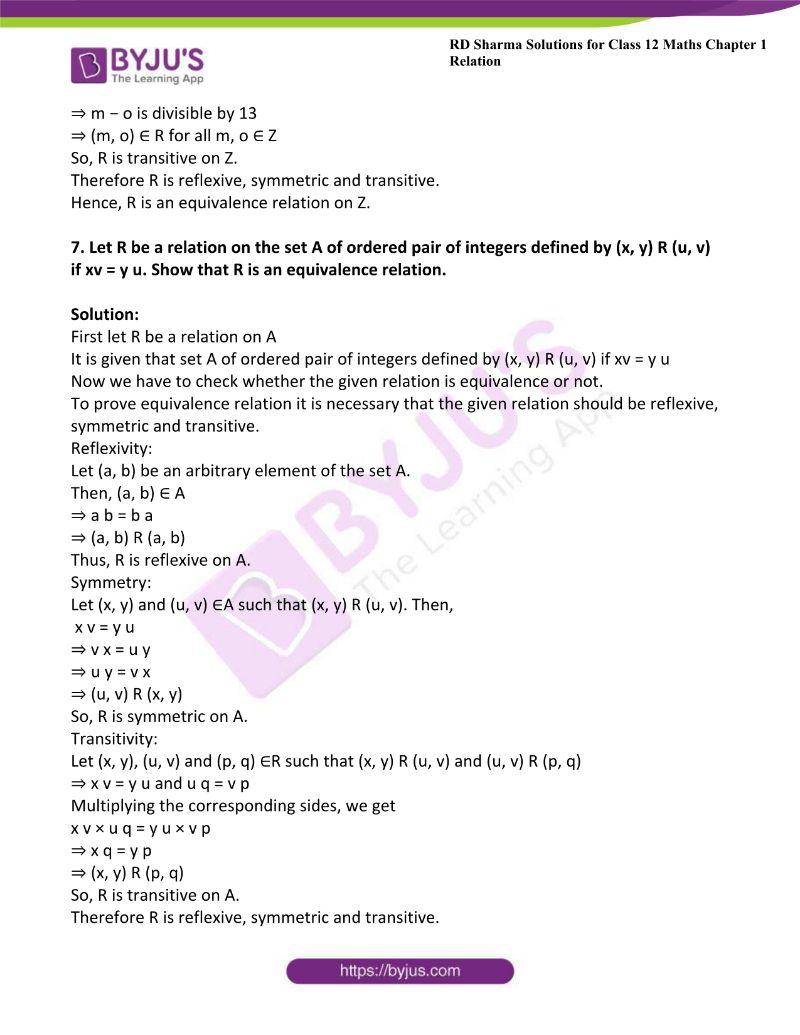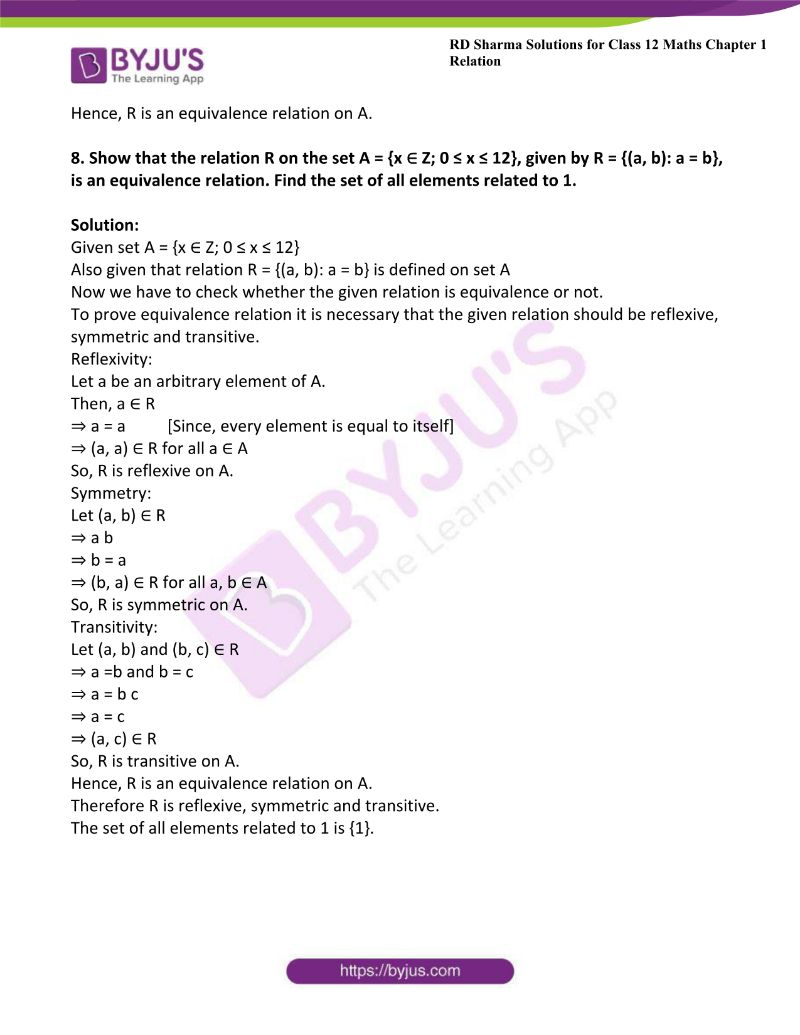### Access another exercise of RD Sharma Solutions For Class 12 Chapter 1 – Relations

Exercise 1.1 Solutions

### Access answers to Maths RD Sharma Solutions For Class 12 Chapter 1 – Relations Exercise 1.2

1. Show that the relation R defined by R = {(a, b): a – b is divisible by 3; a, b ∈ Z} is an equivalence relation.

Solution:

Given R = {(a, b): a – b is divisible by 3; a, b ∈ Z} is a relation

To prove equivalence relation it is necessary that the given relation should be reflexive, symmetric and transitive.

Let us check these properties on R.

Reflexivity:

Let a be an arbitrary element of R.

Then, a – a = 0 = 0 × 3

⇒ a − a is divisible by 3

⇒ (a, a) ∈ R for all a ∈ Z

So, R is reflexive on Z.

Symmetry:

Let (a, b) ∈ R

⇒ a − b is divisible by 3

⇒ a − b = 3p for some p ∈ Z

⇒ b − a = 3 (−p)

Here, −p ∈ Z

⇒ b − a is divisible by 3

⇒ (b, a) ∈ R for all a, b ∈ Z

So, R is symmetric on Z.

Transitivity:

Let (a, b) and (b, c) ∈ R

⇒ a − b and b − c are divisible by 3

⇒ a – b = 3p for some p ∈ Z

And b − c = 3q for some q ∈ Z

Adding the above two equations, we get

a − b + b – c = 3p + 3q

⇒ a − c = 3 (p + q)

Here, p + q ∈ Z

⇒ a − c is divisible by 3

⇒ (a, c) ∈ R for all a, c ∈ Z

So, R is transitive on Z.

Therefore R is reflexive, symmetric and transitive.

Hence, R is an equivalence relation on Z.

2. Show that the relation R on the set Z of integers, given by
R = {(a, b): 2 divides a – b}, is an equivalence relation.

Solution:

Given R = {(a, b): 2 divides a – b} is a relation defined on Z.

To prove equivalence relation it is necessary that the given relation should be reflexive, symmetric and transitive.

Let us check these properties on R.

Reflexivity:

Let a be an arbitrary element of the set Z.

Then, a ∈ R

⇒ a − a = 0 = 0 × 2

⇒ 2 divides a − a

⇒ (a, a) ∈ R for all a ∈ Z

So, R is reflexive on Z.

Symmetry:

Let (a, b) ∈ R

⇒ 2 divides a − b

⇒ (a-b)/2 = p for some p ∈ Z

⇒ (b-a)/2 = – p

Here, −p ∈ Z

⇒ 2 divides b − a

⇒ (b, a) ∈ R for all a, b ∈ Z

So, R is symmetric on Z

Transitivity:

Let (a, b) and (b, c) ∈ R

⇒ 2 divides a−b and 2 divides b−c

⇒ (a-b)/2 = p and (b-c)/2 = q for some p, q ∈ Z

Adding the above two equations, we get

(a – b)/2 + (b – c)/2 = p + q

⇒ (a – c)/2 = p +q

Here, p+ q ∈ Z

⇒ 2 divides a − c

⇒ (a, c) ∈ R for all a, c ∈ Z

So, R is transitive on Z.

Therefore R is reflexive, symmetric and transitive.

Hence, R is an equivalence relation on Z.

3. Prove that the relation R on Z defined by (a, b) ∈ R ⇔ a − b is divisible by 5 is an equivalence relation on Z.

Solution:

Given relation R on Z defined by (a, b) ∈ R ⇔ a − b is divisible by 5

To prove equivalence relation it is necessary that the given relation should be reflexive, symmetric and transitive.

Let us check these properties on R.

Reflexivity:

Let a be an arbitrary element of R. Then,

⇒ a − a = 0 = 0 × 5

⇒ a − a is divisible by 5

⇒ (a, a) ∈ R for all a ∈ Z

So, R is reflexive on Z.

Symmetry:

Let (a, b) ∈ R

⇒ a − b is divisible by 5

⇒ a − b = 5p for some p ∈ Z

⇒ b − a = 5 (−p)

Here, −p ∈ Z                      [Since p ∈ Z]

⇒ b − a is divisible by 5

⇒ (b, a) ∈ R for all a, b ∈ Z

So, R is symmetric on Z.

Transitivity:

Let (a, b) and (b, c) ∈ R

⇒ a − b is divisible by 5

⇒ a − b = 5p for some Z

Also, b − c is divisible by 5

⇒ b − c = 5q for some Z

Adding the above two equations, we get

a −b + b − c = 5p + 5q

⇒ a − c = 5 ( p + q )

⇒ a − c is divisible by 5

Here, p + q ∈ Z

⇒ (a, c) ∈ R for all a, c ∈ Z

So, R is transitive on Z.

Therefore R is reflexive, symmetric and transitive.

Hence, R is an equivalence relation on Z.

4. Let n be a fixed positive integer. Define a relation R on Z as follows:
(a, b) ∈ R ⇔ a − b is divisible by n.
Show that R is an equivalence relation on Z.

Solution:

Given (a, b) ∈ R ⇔ a − b is divisible by n is a relation R defined on Z.

To prove equivalence relation it is necessary that the given relation should be reflexive, symmetric and transitive.

Let us check these properties on R.

Reflexivity:

Let a ∈ N

Here, a − a = 0 = 0 × n

⇒ a − a is divisible by n

⇒ (a, a) ∈ R

⇒ (a, a) ∈ R for all a ∈ Z

So, R is reflexive on Z.

Symmetry:

Let (a, b) ∈ R

Here, a − b is divisible by n

⇒ a − b = n p for some p ∈ Z

⇒ b − a = n (−p)

⇒ b − a is divisible by n                     [ p ∈ Z⇒ − p ∈ Z]

⇒ (b, a) ∈ R

So, R is symmetric on Z.

Transitivity:

Let (a, b) and (b, c) ∈ R

Here, a − b is divisible by n and b − c is divisible by n.

⇒ a − b= n p for some p ∈ Z

And b−c = n q for some q ∈ Z

a – b + b − c = n p + n q

⇒ a − c = n (p + q)

⇒ (a, c) ∈ R for all a, c ∈ Z

So, R is transitive on Z.

Therefore R is reflexive, symmetric and transitive.

Hence, R is an equivalence relation on Z.

5. Let Z be the set of integers. Show that the relation R = {(a, b): a, b ∈ Z and a + b is even} is an equivalence relation on Z.

Solution:

Given R = {(a, b): a, b ∈ Z and a + b is even} is a relation defined on R.

Also given that Z be the set of integers

To prove equivalence relation it is necessary that the given relation should be reflexive, symmetric and transitive.

Let us check these properties on R.

Reflexivity:

Let a be an arbitrary element of Z.

Then, a ∈ R

Clearly, a + a = 2a is even for all a ∈ Z.

⇒ (a, a) ∈ R for all a ∈ Z

So, R is reflexive on Z.

Symmetry:

Let (a, b) ∈ R

⇒ a + b is even

⇒ b + a is even

⇒ (b, a) ∈ R for all a, b ∈ Z

So, R is symmetric on Z.

Transitivity:

Let (a, b) and (b, c) ∈ R

⇒ a + b and b + c are even

Now, let a + b = 2x for some x ∈ Z

And b + c = 2y for some y ∈ Z

Adding the above two equations, we get

A + 2b + c = 2x + 2y

⇒ a + c = 2 (x + y − b), which is even for all x, y, b ∈ Z

Thus, (a, c) ∈ R

So, R is transitive on Z.

Therefore R is reflexive, symmetric and transitive.

Hence, R is an equivalence relation on Z

6. m is said to be related to n if m and n are integers and m − n is divisible by 13. Does this define an equivalence relation?

Solution:

Given that m is said to be related to n if m and n are integers and m − n is divisible by 13

Now we have to check whether the given relation is equivalence or not.

To prove equivalence relation it is necessary that the given relation should be reflexive, symmetric and transitive.

Let R = {(m, n): m, n ∈ Z : m − n is divisible by 13}

Let us check these properties on R.

Reflexivity:

Let m be an arbitrary element of Z.

Then, m ∈ R

⇒ m − m = 0 = 0 × 13

⇒ m − m is divisible by 13

⇒ (m, m) is reflexive on Z.

Symmetry:

Let (m, n) ∈ R.

Then, m − n is divisible by 13

⇒ m − n = 13p

Here, p ∈ Z

⇒ n – m = 13 (−p)

Here, −p ∈ Z

⇒ n − m is divisible by 13

⇒ (n, m) ∈ R for all m, n ∈ Z

So, R is symmetric on Z.

Transitivity:

Let (m, n) and (n, o) ∈R

⇒ m − n and n − o are divisible by 13

⇒ m – n = 13p and n − o = 13q for some p, q ∈ Z

Adding the above two equations, we get

m – n + n − o = 13p + 13q

⇒ m−o = 13 (p + q)

Here, p + q ∈ Z

⇒ m − o is divisible by 13

⇒ (m, o) ∈ R for all m, o ∈ Z

So, R is transitive on Z.

Therefore R is reflexive, symmetric and transitive.

Hence, R is an equivalence relation on Z.

7. Let R be a relation on the set A of ordered pair of integers defined by (x, y) R (u, v) if xv = y u. Show that R is an equivalence relation.

Solution:

First let R be a relation on A

It is given that set A of ordered pair of integers defined by (x, y) R (u, v) if xv = y u

Now we have to check whether the given relation is equivalence or not.

To prove equivalence relation it is necessary that the given relation should be reflexive, symmetric and transitive.

Reflexivity:

Let (a, b) be an arbitrary element of the set A.

Then, (a, b) ∈ A

⇒ a b = b a

⇒ (a, b) R (a, b)

Thus, R is reflexive on A.

Symmetry:

Let (x, y) and (u, v) ∈A such that (x, y) R (u, v). Then,

x v = y u

⇒ v x = u y

⇒ u y = v x

⇒ (u, v) R (x, y)

So, R is symmetric on A.

Transitivity:

Let (x, y), (u, v) and (p, q) ∈R such that (x, y) R (u, v) and (u, v) R (p, q)

⇒ x v = y u and u q = v p

Multiplying the corresponding sides, we get

x v × u q = y u × v p

⇒ x q = y p

⇒ (x, y) R (p, q)

So, R is transitive on A.

Therefore R is reflexive, symmetric and transitive.

Hence, R is an equivalence relation on A.

8. Show that the relation R on the set A = {x ∈ Z; 0 ≤ x ≤ 12}, given by R = {(a, b): a = b}, is an equivalence relation. Find the set of all elements related to 1.

Solution:

Given set A = {x ∈ Z; 0 ≤ x ≤ 12}

Also given that relation R = {(a, b): a = b} is defined on set A

Now we have to check whether the given relation is equivalence or not.

To prove equivalence relation it is necessary that the given relation should be reflexive, symmetric and transitive.

Reflexivity:

Let a be an arbitrary element of A.

Then, a ∈ R

⇒ a = a          [Since, every element is equal to itself]

⇒ (a, a) ∈ R for all a ∈ A

So, R is reflexive on A.

Symmetry:

Let (a, b) ∈ R

⇒ a b

⇒ b = a

⇒ (b, a) ∈ R for all a, b ∈ A

So, R is symmetric on A.

Transitivity:

Let (a, b) and (b, c) ∈ R

⇒ a =b and b = c

⇒ a = b c

⇒ a = c

⇒ (a, c) ∈ R

So, R is transitive on A.

Hence, R is an equivalence relation on A.

Therefore R is reflexive, symmetric and transitive.

The set of all elements related to 1 is {1}.